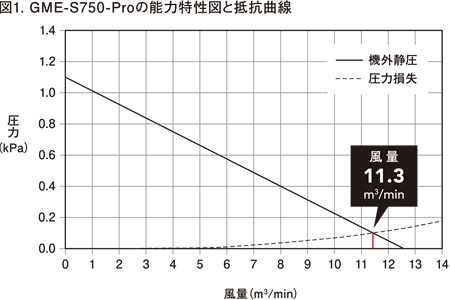# Choosing a mist collector

Those requesting for model selection via the Web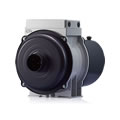By entering the detailed information for the model selection,
the calculations will be carried out automatically to select a model that matches the site.

Those who are considering product introductionPlease feel free to contact us for inquiries such as on-site confirmation of product introduction, product details and prices.

## Choosing a mist collector

The mist collector selects the model based on the required air volume. Check the required air volume in the following order.

### In case of high airtightness for　the source of mist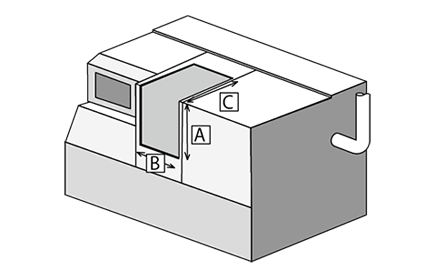Q = (A×B×C)×N
 Q： Necessary amount of throughput　for the mist collector (m³/min) (A×B×C)： Volume of processing room （m³） N： Ventilation frequency per minute （N/min） Ventilation frequency is 4 to 8 times and　is set arbitrarily.

### In case of low airtightness for the source of mist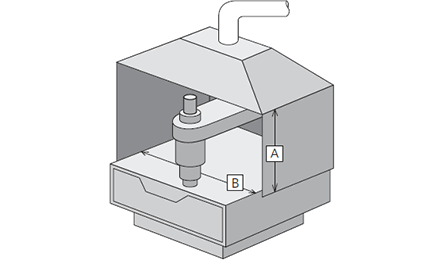Q = A×B×V×60
 Q： Necessary amount of throughput for the mist collector (m³/min) A×B： The opening area (m²) V： Wind velocity (m/sec)　(=0.5m/sec)

### Samples for the model selection (In case of High airtightness for the source of mist)

When selecting a mist collector to be mounted on an NC lathe machine with A = 1000 mm, B = 1000 mm, C = 1000 mm with a ventilation frequency of 8 times.

#### (1) Calculate the necessary amount of throughput for the mist collector.

Q = 1×1×1×8 = 8.0(m³/min)

#### (2) Select a mist collector temporally.

Temporally select a model that has the maximum air capacity more than the necessary amount of throughput in (1) . In this case, select GME-S750 with 11m³ /min of the maximum amount of throughput.

#### (3) Calculate the length of straight pipe parts.

1.0 + 1.0 + 0.5 = 2.5(m)

#### (4) Convert the relevant length of a straight pipe　for the curved part from Table1.

As R/D of the curved part is 1.5, the relevant length of a straight pipe is　10D based on Table1. As there are 2 parts of the curved part and the　discharge opening of GME-S750 selected temporally in (2) is φ125, the　relevant length of the straight pipe for the curved parts of ducts can be　calculated as follows.

10×0.125×2 = 2.5(m)

#### (5) Calculate total length of the straight pipes.

2.5 + 2.5 = 5.0(m)

#### (6) Select the mist collector.

Check the cross point of the resistance curb for (5) the total length of the straight pipes and (2) the P-Q curve of the temporally-selected mist collector (Figure1).
According to Figure1, the air volume is 9.5m /min for 60Hz when the relevant length of the straight pipe for ducts is 5m, which exceeds the necessary amount of throughput calculated in (1) . Thus, select GME-S750.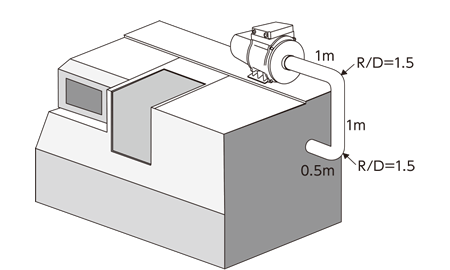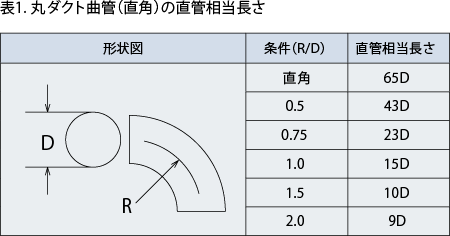*Values may change due to the type of ducts. Please ask details to a duct maker.